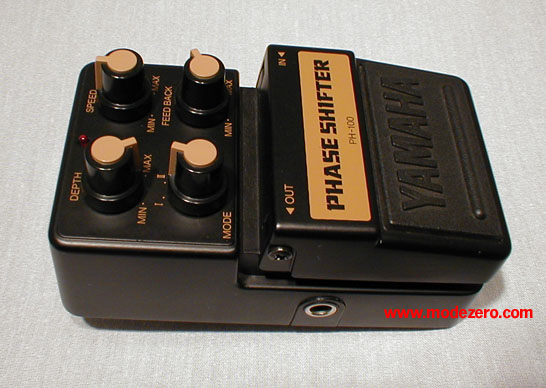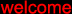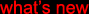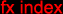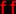(c) 2000-2009

mode zero

yamaha ph-100 phase shifter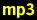guitar, no effectguitar, mode = I, depth = max, speed = 1/2, feedback = 1/2guitar, mode = I, depth = max, speed = near max, feedback = maxguitar, mode = II, depth = 2/3, speed = 1/3, feedback = maxguitar, mode = II, depth = max, speed = 2/3, feedback = maxmatrix-1000 pad, no effectmatrix-1000 pad, mode = I, depth = max, speed = 1/3, feedback = maxmatrix-1000 pad, mode = II, depth = max, speed = 1/3, feedback = maxmatrix-1000 pad, mode = II, depth = 1/2, speed = near max, feedback = 1/4